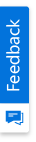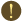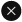We use cookies to give you the best experience on our website. If you continue to browse, then you agree to our privacy policy and cookie policy.Unfortunately, activation email could not send to your email. Please try again.# How to check if a cell in a Grid is a formula cell or not?

Platform: WinForms |
Tags:

The custom server has implementation to detect a formula cell with formula and value in it. IsFormulaCell is the function that servers the information for a given row and column index. This function points out the formula used in that cell and the result of the formula. This returns false when the cell is not a formula cell.

The syntax for this function is,

Where, row is the index of the row, col is the index of the column, formula retrieves the formula of the cell, computedValue retrieves the computed or calculated value of the cell, IsFormulaCell returns true when the given row and col is a FormulaCell, false otherwise.

The sample script is as follows.

QTP script

 SwfWindow("GridControl").Move 592,516 SwfWindow("GridControl").SwfObject("gridControl1").SetCurrentCell 8,4 SwfWindow("GridControl").SwfObject("gridControl1").SetCellData 8,4,"40" row = 8 IsFormula = false formula = "" result ="" For col = 5 To 8 IsFormula = SwfWindow("GridControl").SwfObject("gridControl1").IsFormulaCell(row, col, formula, result) If IsFormula Then MsgBox ("The cell "&row &", "& col &" is a Formula Cell, with Formula "& formula&" and results "& result) End If NextNote: Run the above script in the QTP server.

2X faster development

The ultimate WinForms UI toolkit to boost your development speed.You are using an outdated version of Internet Explorer that may not display all features of this and other websites. Upgrade to Internet Explorer 8 or newer for a better experience.